Chapter 18 The Stars: A Celestial Census

# 18.2 Measuring Stellar Masses

### Learning Objectives

By the end of this section, you will be able to:

• Distinguish the different types of binary star systems
• Understand how we can apply Newton’s version of Kepler’s third law to derive the sum of star masses in a binary star system
• Apply the relationship between stellar mass and stellar luminosity to determine the physical characteristics of a star

The mass of a star—how much material it contains—is one of its most important characteristics. If we know a star’s mass, as we shall see, we can estimate how long it will shine and what its ultimate fate will be. Yet the mass of a star is very difficult to measure directly. Somehow, we need to put a star on the cosmic equivalent of a scale.

Luckily, not all stars live like the Sun, in isolation from other stars. About half the stars are binary stars—two stars that orbit each other, bound together by gravity. Masses of binary stars can be calculated from measurements of their orbits, just as the mass of the Sun can be derived by measuring the orbits of the planets around it (see Orbits and Gravity).

# Binary Stars

Before we discuss in more detail how mass can be measured, we will take a closer look at stars that come in pairs. The first binary star was discovered in 1650, less than half a century after Galileo began to observe the sky with a telescope. John Baptiste Riccioli (1598–1671), an Italian astronomer, noted that the star Mizar, in the middle of the Big Dipper’s handle, appeared through his telescope as two stars. Since that discovery, thousands of binary stars have been cataloged. (Astronomers call any pair of stars that appear to be close to each other in the sky double stars, but not all of these form a true binary, that is, not all of them are physically associated. Some are just chance alignments of stars that are actually at different distances from us.) Although stars most commonly come in pairs, there are also triple and quadruple systems.

One well-known binary star is Castor, located in the constellation of Gemini. By 1804, astronomer William Herschel, who also discovered the planet Uranus, had noted that the fainter component of Castor had slightly changed its position relative to the brighter component. (We use the term “component” to mean a member of a star system.) Here was evidence that one star was moving around another. It was actually the first evidence that gravitational influences exist outside the solar system. The orbital motion of a binary star is shown in Figure 1. A binary star system in which both of the stars can be seen with a telescope is called a visual binary.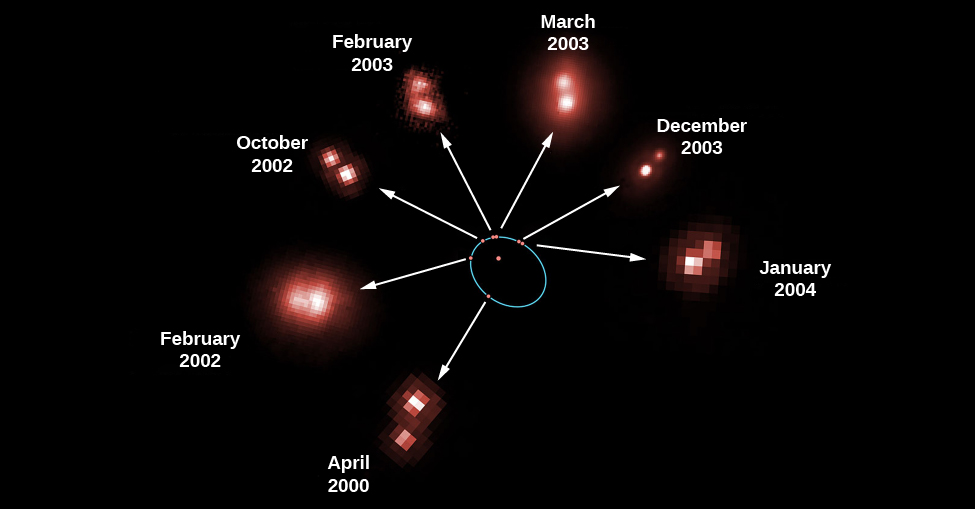Figure 1. This figure shows seven observations of the mutual revolution of two stars, one a brown dwarf and one an ultra-cool L dwarf. Each red dot on the orbit, which is shown by the blue ellipse, corresponds to the position of one of the dwarfs relative to the other. The reason that the pair of stars looks different on the different dates is that some images were taken with the Hubble Space Telescope and others were taken from the ground. The arrows point to the actual observations that correspond to the positions of each red dot. From these observations, an international team of astronomers directly measured the mass of an ultra-cool brown dwarf star for the first time. Barely the size of the planet Jupiter, the dwarf star weighs in at just 8.5% of the mass of our Sun. (credit: modification of work by ESA/NASA and Herve Bouy (Max-Planck-Institut für Extraterrestrische Physik/ESO, Germany))

Edward C. Pickering (1846–1919), at Harvard, discovered a second class of binary stars in 1889—a class in which only one of the stars is actually seen directly. He was examining the spectrum of Mizar and found that the dark absorption lines in the brighter star’s spectrum were usually double. Not only were there two lines where astronomers normally saw only one, but the spacing of the lines was constantly changing. At times, the lines even became single. Pickering correctly deduced that the brighter component of Mizar, called Mizar A, is itself really two stars that revolve about each other in a period of 104 days. A star like Mizar A, which appears as a single star when photographed or observed visually through the telescope, but which spectroscopy shows really to be a double star, is called a spectroscopic binary.

Mizar, by the way, is a good example of just how complex such star systems can be. Mizar has been known for centuries to have a faint companion called Alcor, which can be seen without a telescope. Mizar and Alcor form an optical double—a pair of stars that appear close together in the sky but do not orbit each other. Through a telescope, as Riccioli discovered in 1650, Mizar can be seen to have another, closer companion that does orbit it; Mizar is thus a visual binary. The two components that make up this visual binary, known as Mizar A and Mizar B, are both spectroscopic binaries. So, Mizar is really a quadruple system of stars.

Strictly speaking, it is not correct to describe the motion of a binary star system by saying that one star orbits the other. Gravity is a mutual attraction. Each star exerts a gravitational force on the other, with the result that both stars orbit a point between them called the centre of mass. Imagine that the two stars are seated at either end of a seesaw. The point at which the fulcrum would have to be located in order for the seesaw to balance is the centre of mass, and it is always closer to the more massive star as illustrated in Figure 2.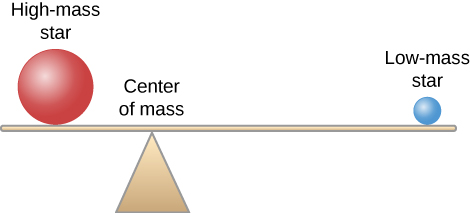Figure 2. In a binary star system, both stars orbit their centre of mass. The image shows the relative positions of two, different-mass stars from their centre of mass, similar to how two masses would have to be located on a seesaw in order to keep it level. The star with the higher mass will be found closer to the centre of mass, while the star with the lower mass will be farther from it.

Figure 3 shows two stars (A and B) moving around their centre of mass, along with one line in the spectrum of each star that we observe from the system at different times. When one star is approaching us relative to the centre of mass, the other star is receding from us. In the top left illustration, star A is moving toward us, so the line in its spectrum is Doppler-shifted toward the blue end of the spectrum. Star B is moving away from us, so its line shows a redshift. When we observe the composite spectrum of the two stars, the line appears double. When the two stars are both moving across our line of sight (neither away from nor toward us), they both have the same radial velocity (that of the pair’s centre of mass); hence, the spectral lines of the two stars come together. This is shown in the two bottom illustrations of Figure 3.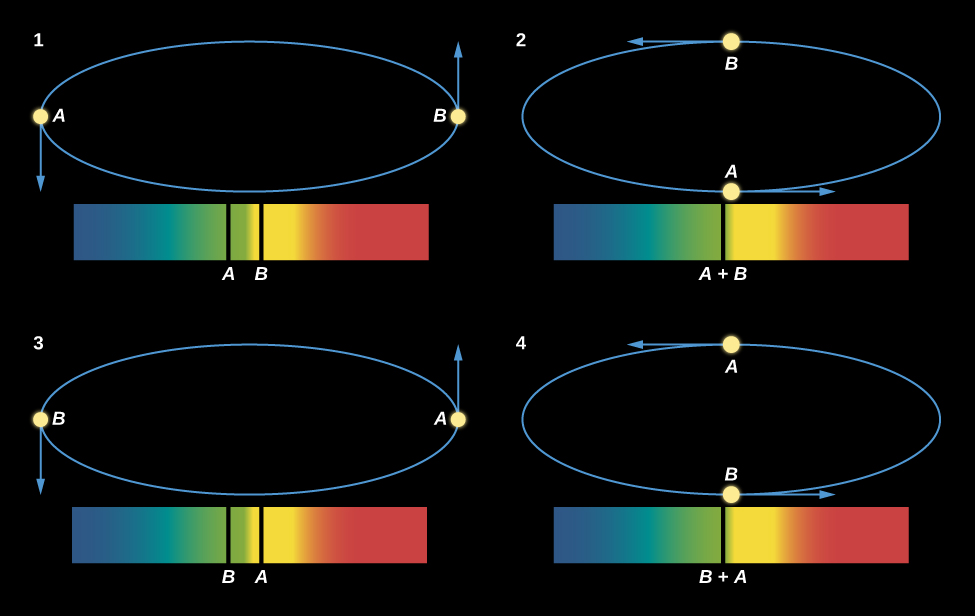Figure 3. We see changes in velocity because when one star is moving toward Earth, the other is moving away; half a cycle later, the situation is reversed. Doppler shifts cause the spectral lines to move back and forth. In diagrams 1 and 3, lines from both stars can be seen well separated from each other. When the two stars are moving perpendicular to our line of sight (that is, they are not moving either toward or away from us), the two lines are exactly superimposed, and so in diagrams 2 and 4, we see only a single spectral line. Note that in the diagrams, the orbit of the star pair is tipped slightly with respect to the viewer (or if the viewer were looking at it in the sky, the orbit would be tilted with respect to the viewer’s line of sight). If the orbit were exactly in the plane of the page or screen (or the sky), then it would look nearly circular, but we would see no change in radial velocity (no part of the motion would be toward us or away from us.) If the orbit were perpendicular to the plane of the page or screen, then the stars would appear to move back and forth in a straight line, and we would see the largest-possible radial velocity variations.

A plot showing how the velocities of the stars change with time is called a radial velocity curve; the curve for the binary system in Figure 3 is shown in Figure 4 below.

# Masses from the Orbits of Binary Stars

We can estimate the masses of binary star systems using Newton’s reformulation of Kepler’s third law (discussed in Newton’s Universal Law of Gravitation). Kepler found that the time a planet takes to go around the Sun is related by a specific mathematical formula to its distance from the Sun. In our binary star situation, if two objects are in mutual revolution, then the period (P) with which they go around each other is related to the semimajor axis (D) of the orbit of one with respect to the other, according to this equation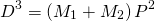where D is in astronomical units, P is measured in years, and M1 + M2 is the sum of the masses of the two stars in units of the Sun’s mass. This is a very useful formula for astronomers; it says that if we can observe the size of the orbit and the period of mutual revolution of the stars in a binary system, we can calculate the sum of their masses.

Most spectroscopic binaries have periods ranging from a few days to a few months, with separations of usually less than 1 AU between their member stars. Recall that an AU is the distance from Earth to the Sun, so this is a small separation and very hard to see at the distances of stars. This is why many of these systems are known to be double only through careful study of their spectra.

We can analyze a radial velocity curve, such as the one in Figure 4, to determine the masses of the stars in a spectroscopic binary. This is complex in practice but not hard in principle. We measure the speeds of the stars from the Doppler effect. We then determine the period—how long the stars take to go through an orbital cycle—from the velocity curve. Knowing how fast the stars are moving and how long they take to go around tells us the circumference of the orbit and, hence, the separation of the stars in kilometres or astronomical units. From Kepler’s law, the period and the separation allow us to calculate the sum of the stars’ masses.

Of course, knowing the sum of the masses is not as useful as knowing the mass of each star separately. But the relative orbital speeds of the two stars can tell us how much of the total mass each star has. As we saw in our seesaw analogy, the more massive star is closer to the centre of mass and therefore has a smaller orbit. Therefore, it moves more slowly to get around in the same time compared to the more distant, lower-mass star. If we sort out the speeds relative to each other, we can sort out the masses relative to each other. In practice, we also need to know how the binary system is oriented in the sky to our line of sight, but if we do, and the just-described steps are carried out carefully, the result is a calculation of the masses of each of the two stars in the system.

To summarize, a good measurement of the motion of two stars around a common centre of mass, combined with the laws of gravity, allows us to determine the masses of stars in such systems. These mass measurements are absolutely crucial to developing a theory of how stars evolve. One of the best things about this method is that it is independent of the location of the binary system. It works as well for stars 100 light-years away from us as for those in our immediate neighbourhood.

To take a specific example, Sirius is one of the few binary stars in Appendix for which we have enough information to apply Kepler’s third law:In this case, the two stars, the one we usually call Sirius and its very faint companion, are separated by about 20 AU and have an orbital period of about 50 years. If we place these values in the formula we would have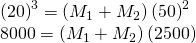This can be solved for the sum of the masses: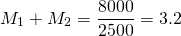Therefore, the sum of masses of the two stars in the Sirius binary system is 3.2 times the Sun’s mass. In order to determine the individual mass of each star, we would need the velocities of the two stars and the orientation of the orbit relative to our line of sight.

# The Range of Stellar Masses

How large can the mass of a star be? Stars more massive than the Sun are rare. None of the stars within 30 light-years of the Sun has a mass greater than four times that of the Sun. Searches at large distances from the Sun have led to the discovery of a few stars with masses up to about 100 times that of the Sun, and a handful of stars (a few out of several billion) may have masses as large as 250 solar masses. However, most stars have less mass than the Sun.

According to theoretical calculations, the smallest mass that a true star can have is about 1/12 that of the Sun. By a “true” star, astronomers mean one that becomes hot enough to fuse protons to form helium (as discussed in The Sun: A Nuclear Powerhouse). Objects with masses between roughly 1/100 and 1/12 that of the Sun may produce energy for a brief time by means of nuclear reactions involving deuterium, but they do not become hot enough to fuse protons. Such objects are intermediate in mass between stars and planets and have been given the name brown dwarfs as pictured in Figure 5. Brown dwarfs are similar to Jupiter in radius but have masses from approximately 13 to 80 times larger than the mass of Jupiter.1

Still-smaller objects with masses less than about 1/100 the mass of the Sun (or 10 Jupiter masses) are called planets. They may radiate energy produced by the radioactive elements that they contain, and they may also radiate heat generated by slowly compressing under their own weight (a process called gravitational contraction). However, their interiors will never reach temperatures high enough for any nuclear reactions, to take place. Jupiter, whose mass is about 1/1000 the mass of the Sun, is unquestionably a planet, for example. Until the 1990s, we could only detect planets in our own solar system, but now we have thousands of them elsewhere as well. (We will discuss these exciting observations in The Birth of Stars and the Discovery of Planets outside the Solar System.)

# The Mass-Luminosity Relation

Now that we have measurements of the characteristics of many different types of stars, we can search for relationships among the characteristics. For example, we can ask whether the mass and luminosity of a star are related. It turns out that for most stars, they are: The more massive stars are generally also the more luminous. This relationship, known as the mass-luminosity relation, is shown graphically in Figure 6. Each point represents a star whose mass and luminosity are both known. The horizontal position on the graph shows the star’s mass, given in units of the Sun’s mass, and the vertical position shows its luminosity in units of the Sun’s luminosity.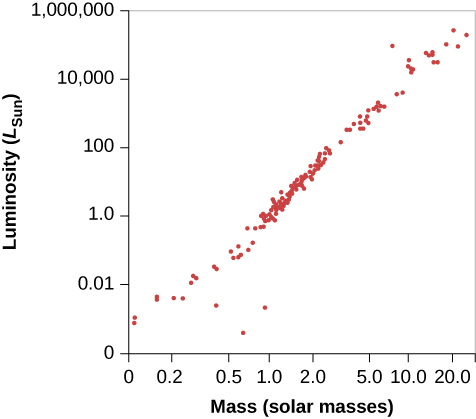Figure 6. The plotted points show the masses and luminosities of stars. The three points lying below the sequence of points are all white dwarf stars.

We can also say this in mathematical terms.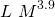It’s a reasonably good approximation to say that luminosity (expressed in units of the Sun’s luminosity) varies as the fourth power of the mass (in units of the Sun’s mass). (The symbol ~ means the two quantities are proportional.) If two stars differ in mass by a factor of 2, then the more massive one will be 24, or about 16 times brighter; if one star is 1/3 the mass of another, it will be approximately 81 times less luminous.

Calculating the Mass from the Luminosity of a Star
The mass-luminosity formula can be rewritten so that a value of mass can be determined if the luminosity is known.

Solution
First, we must get our units right by expressing both the mass and the luminosity of a star in units of the Sun’s mass and luminosity: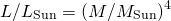Now we can take the 4th root of both sides, which is equivalent to taking both sides to the 1/4 = 0.25 power. The formula in this case would be: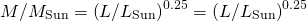In the previous section, we determined the sum of the masses of the two stars in the Sirius binary system (Sirius and its faint companion) using Kepler’s third law to be 3.2 solar masses. Using the mass-luminosity relationship, calculate the mass of each individual star.

In Appendix J, Sirius is listed with a luminosity 23 times that of the Sun. This value can be inserted into the mass-luminosity relationship to get the mass of Sirius: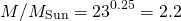The mass of the companion star to Sirius is then 3.2 – 2.2 = 1.0 solar mass.

Notice how good this mass-luminosity relationship is. Most stars, as shown in Figure 6, fall along a line running from the lower-left (low mass, low luminosity) corner of the diagram to the upper-right (high mass, high luminosity) corner. About 90% of all stars obey the mass-luminosity relation. Later, we will explore why such a relationship exists and what we can learn from the roughly 10% of stars that “disobey” it.

# Key Concepts and Summary

The masses of stars can be determined by analysis of the orbit of binary stars—two stars that orbit a common centre of mass. In visual binaries, the two stars can be seen separately in a telescope, whereas in a spectroscopic binary, only the spectrum reveals the presence of two stars. Stellar masses range from about 1/12 to more than 100 times the mass of the Sun (in rare cases, going to 250 times the Sun’s mass). Objects with masses between 1/12 and 1/100 that of the Sun are called brown dwarfs. Objects in which no nuclear reactions can take place are planets. The most massive stars are, in most cases, also the most luminous, and this correlation is known as the mass-luminosity relation.

## Footnotes

1. 1 Exactly where to put the dividing line between planets and brown dwarfs is a subject of some debate among astronomers as we write this book (as is, in fact, the exact definition of each of these objects). Even those who accept deuterium fusion (see The Birth of Stars and the Discovery of Planets outside the Solar System) as the crucial issue for brown dwarfs concede that, depending on the composition of the star and other factors, the lowest mass for such a dwarf could be anywhere from 11 to 16 Jupiter masses.

## Glossary

binary stars
two stars that revolve about each other
brown dwarf
an object intermediate in size between a planet and a star; the approximate mass range is from about 1/100 of the mass of the Sun up to the lower mass limit for self-sustaining nuclear reactions, which is about 1/12 the mass of the Sun
mass-luminosity relation
the observed relation between the masses and luminosities of many (90% of all) stars
spectroscopic binary
a binary star in which the components are not resolved but whose binary nature is indicated by periodic variations in radial velocity, indicating orbital motion
visual binary
a binary star in which the two components are telescopically resolved